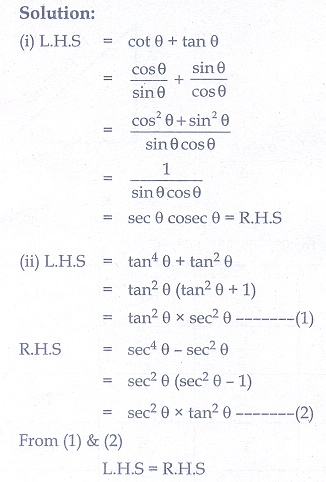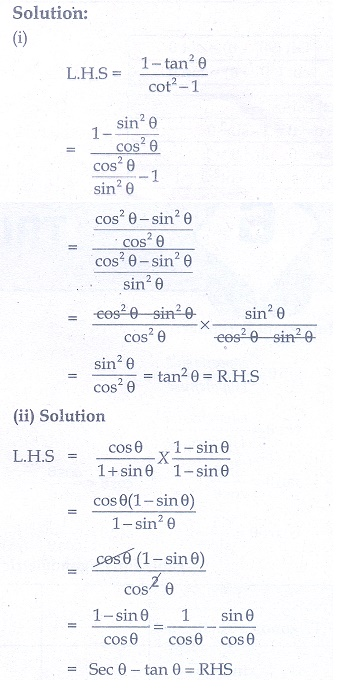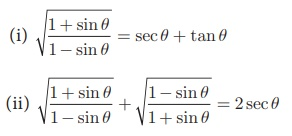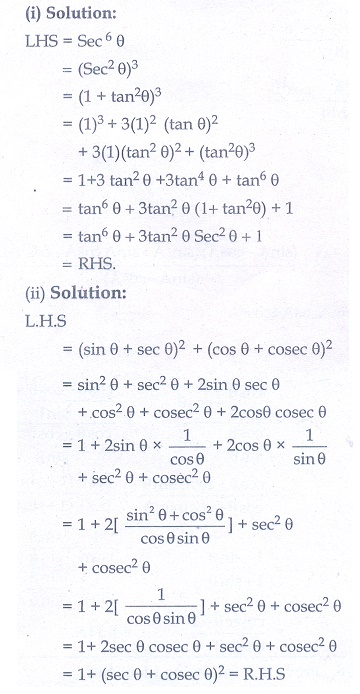Home | | Maths 10th Std | Exercise 6.1: Trigonometric identities

# Exercise 6.1: Trigonometric identities

Maths Book back answers and solution for Exercise questions - Mathematics : Trigonometry: Trigonometric identities: Exercise Problem Questions with Answer

### Exercise 6.1

1. Prove the following identities.

(i) cot θ + tan θ = sec θ cosec θ

(ii) tan 4 θ + tan2 θ = sec 4 θ − sec2 θ2. Prove the following identities.3. Prove the following identities.4. Prove the following identities.

(i) sec 6 θ = tan6 θ + 3 tan 2 θ sec2 θ +1

(ii) (sin θ + sec θ)2  + (cos θ + cosec θ)2  = 1 + (sec θ + cosec θ)25. Prove the following identities.

(i) sec 4 θ (1 − sin 4 θ) −2 tan2 θ = 1

(ii)6. Prove the following identities.7. (i) If sin θ + cos θ = √3, then prove that tan θ + cot θ = 1.

(ii) If √3sinθ − cosθ = 0, then show that tan 3θ = (3 tan θ − tan3θ) / (1 − 3 tan2 θ)8. (i) If, then prove that (m2 + n2 )cos2 β = n2

(ii) If cot θ + tan θ = x and sec θ − cos θ = y , then prove that (x 2y)2/3 – (xy2)2/3 = 19. (i) If sin θ + cos θ = p and sec θ + cosec θ = q , then prove that q ( p2 − 1) = 2p

(ii) If sin θ(1 + sin2 θ) = cos2 θ , then prove that cos6 θ − 4 cos4 θ + 8 cos2 θ = 410. IfTags : Problem Questions with Answer, Solution | Mathematics , 10th Mathematics : UNIT 6 : Trigonometry
Study Material, Lecturing Notes, Assignment, Reference, Wiki description explanation, brief detail
10th Mathematics : UNIT 6 : Trigonometry : Exercise 6.1: Trigonometric identities | Problem Questions with Answer, Solution | Mathematics